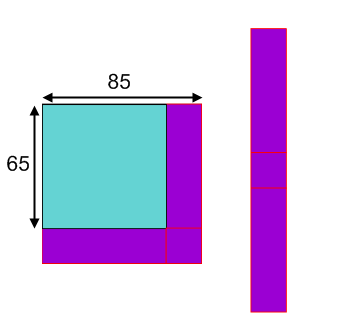#### You may also like### Euler's Squares

Euler found four whole numbers such that the sum of any two of the numbers is a perfect square...### DOTS Division

Take any pair of two digit numbers x=ab and y=cd where, without loss of generality, ab > cd . Form two 4 digit numbers r=abcd and s=cdab and calculate: {r^2 - s^2} /{x^2 - y^2}.### 2-digit Square

A 2-Digit number is squared. When this 2-digit number is reversed and squared, the difference between the squares is also a square. What is the 2-digit number?

# Plus Minus

##### Age 14 to 16 Challenge Level:
Look at the diagram below:Which part of the diagram represents $85^2$?
Which part represents $65^2$?
Which part represents $85^2-65^2$?